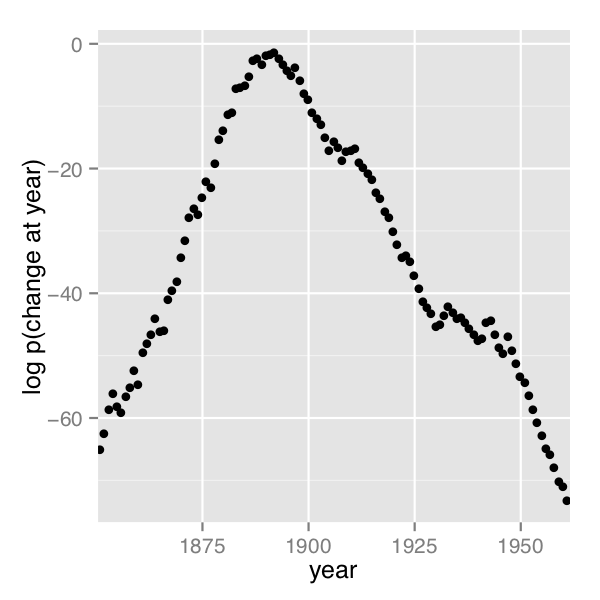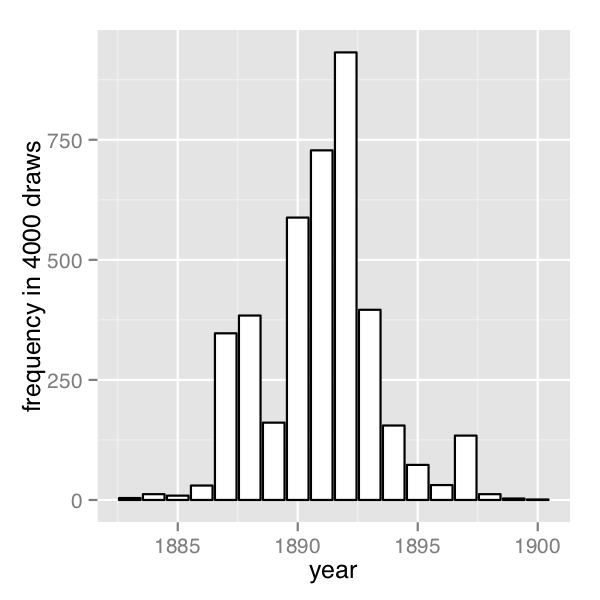This is an old version, view current version.

## 7.2 Change Point Models

The first example is a model of coal mining disasters in the U.K. for the years 1851–1962.16

### Model with Latent Discrete Parameter

Fonnesbeck et al. (2013, Section 3.1) provides a Poisson model of disaster $$D_t$$ in year $$t$$ with two rate parameters, an early rate ($$e$$) and late rate ($$l$$), that change at a given point in time $$s$$. The full model expressed using a latent discrete parameter $$s$$ is \begin{align*} e &\sim \textsf{exponential}(r_e) \\ l &\sim \textsf{exponential}(r_l) \\ s &\sim \textsf{uniform}(1, T) \\ D_t &\sim \textsf{Poisson}(t < s \; ? \; e \: : \: l) \end{align*}

The last line uses the conditional operator (also known as the ternary operator), which is borrowed from C and related languages. The conditional operator has the same behavior as its counterpart in C++.17

It uses a compact notation involving separating its three arguments by a question mark (?) and a colon (:). The conditional operator is defined by $c \; ? \; x_1 \: : \: x_2 = \begin{cases} \ x_1 & \quad\text{if } c \text{ is true (i.e., non-zero), and} \\ \ x_2 & \quad\text{if } c \text{ is false (i.e., zero).} \end{cases}$

### Marginalizing out the Discrete Parameter

To code this model in Stan, the discrete parameter $$s$$ must be marginalized out to produce a model defining the log of the probability function $$p(e,l,D_t)$$. The full joint probability factors as \begin{align*} p(e,l,s,D) &= p(e) \, p(l) \, p(s) \, p(D \mid s, e, l) \\ &= \textsf{exponential}(e \mid r_e) \ \textsf{exponential}(l \mid r_l) \ \textsf{uniform}(s \mid 1, T) \\ &\qquad \prod_{t=1}^T \textsf{Poisson}(D_t \mid t < s \; ? \; e \: : \: l), \end{align*}

To marginalize, an alternative factorization into prior and likelihood is used, $p(e,l,D) = p(e,l) \, p(D \mid e,l),$ where the likelihood is defined by marginalizing $$s$$ as \begin{align*} p(D \mid e,l) &= \sum_{s=1}^T p(s, D \mid e,l) \\ &= \sum_{s=1}^T p(s) \, p(D \mid s,e,l) \\ &= \sum_{s=1}^T \textsf{uniform}(s \mid 1,T) \, \prod_{t=1}^T \textsf{Poisson}(D_t \mid t < s \; ? \; e \: : \: l). \end{align*}

Stan operates on the log scale and thus requires the log likelihood, \begin{align*} \log p(D \mid e,l) &= \texttt{log}\mathtt{\_}\texttt{sum}\mathtt{\_}\texttt{exp}_{s=1}^T \left( \log \textsf{uniform}(s \mid 1, T) \vphantom{\sum_{t=1}^T}\right. \\ &\qquad \left. + \sum_{t=1}^T \log \textsf{Poisson}(D_t \mid t < s \; ? \; e \: : \: l) \right), \end{align*} where the log sum of exponents function is defined by $\texttt{log}\mathtt{\_}\texttt{sum}\mathtt{\_}\texttt{exp}_{n=1}^N \, \alpha_n = \log \sum_{n=1}^N \exp(\alpha_n).$

The log sum of exponents function allows the model to be coded directly in Stan using the built-in function log_sum_exp, which provides both arithmetic stability and efficiency for mixture model calculations.

### Coding the Model in Stan

The Stan program for the change point model is shown in the figure below. The transformed parameter lp[s] stores the quantity $$\log p(s, D \mid e, l)$$.

data {
real<lower=0> r_e;
real<lower=0> r_l;

int<lower=1> T;
int<lower=0> D[T];
}
transformed data {
real log_unif;
log_unif = -log(T);
}
parameters {
real<lower=0> e;
real<lower=0> l;
}
transformed parameters {
vector[T] lp;
lp = rep_vector(log_unif, T);
for (s in 1:T)
for (t in 1:T)
lp[s] = lp[s] + poisson_lpmf(D[t] | t < s ? e : l);
}
model {
e ~ exponential(r_e);
l ~ exponential(r_l);
target += log_sum_exp(lp);
}

A change point model in which disaster rates D[t] have one rate, e, before the change point and a different rate, l, after the change point. The change point itself, s, is marginalized out as described in the text.

Although the change-point model is coded directly, the doubly nested loop used for s and t is quadratic in T. Luke Wiklendt pointed out that a linear alternative can be achieved by the use of dynamic programming similar to the forward-backward algorithm for Hidden Markov models; he submitted a slight variant of the following code to replace the transformed parameters block of the above Stan program.

transformed parameters {
vector[T] lp;
{
vector[T + 1] lp_e;
vector[T + 1] lp_l;
lp_e = 0;
lp_l = 0;
for (t in 1:T) {
lp_e[t + 1] = lp_e[t] + poisson_lpmf(D[t] | e);
lp_l[t + 1] = lp_l[t] + poisson_lpmf(D[t] | l);
}
lp = rep_vector(log_unif + lp_l[T + 1], T)
}
}

As should be obvious from looking at it, it has linear complexity in T rather than quadratic. The result for the mining-disaster data is about 20 times faster; the improvement will be greater for larger T.

The key to understanding Wiklendt’s dynamic programming version is to see that head(lp_e) holds the forward values, whereas lp_l[T + 1] - head(lp_l, T) holds the backward values; the clever use of subtraction allows lp_l to be accumulated naturally in the forward direction.

### Fitting the Model with MCMC

This model is easy to fit using MCMC with NUTS in its default configuration. Convergence is fast and sampling produces roughly one effective sample every two iterations. Because it is a relatively small model (the inner double loop over time is roughly 20,000 steps), it is fast.

The value of lp for each iteration for each change point is available because it is declared as a transformed parameter. If the value of lp were not of interest, it could be coded as a local variable in the model block and thus avoid the I/O overhead of saving values every iteration.

### Posterior Distribution of the Discrete Change Point

The value of lp[s] in a given iteration is given by $$\log p(s,D \mid e,l)$$ for the values of the early and late rates, $$e$$ and $$l$$, in the iteration. In each iteration after convergence, the early and late disaster rates, $$e$$ and $$l$$, are drawn from the posterior $$p(e,l \mid D)$$ by MCMC sampling and the associated lp calculated. The value of lp may be normalized to calculate $$p(s \mid e,l,D)$$ in each iteration, based on on the current values of $$e$$ and $$l$$. Averaging over iterations provides an unnormalized probability estimate of the change point being $$s$$ (see below for the normalizing constant), \begin{align*} p(s \mid D) &\propto q(s \mid D) \\ &= \frac{1}{M} \sum_{m=1}^{M} \exp(\texttt{lp}[m,s]). \end{align*} where $$\texttt{lp}[m,s]$$ represents the value of lp in posterior draw $$m$$ for change point $$s$$. By averaging over draws, $$e$$ and $$l$$ are themselves marginalized out, and the result has no dependence on a given iteration’s value for $$e$$ and $$l$$. A final normalization then produces the quantity of interest, the posterior probability of the change point being $$s$$ conditioned on the data $$D$$, $p(s \mid D) = \frac{q(s \mid D)}{\sum_{s'=1}^T q(s' \mid D)}.$

A plot of the values of $$\log p(s \mid D)$$ computed using Stan 2.4’s default MCMC implementation is shown in the posterior plot.

Log probability of change point being in year, calculated analytically.Figure 7.1: Analytical change-point posterior

The frequency of change points generated by sampling the discrete change points.Figure 7.2: Sampled change-point posterior

In order their range of estimates be visible, the first plot is on the log scale and the second plot on the linear scale; note the narrower range of years in the second plot resulting from sampling. The posterior mean of $$s$$ is roughly 1891.

### Discrete Sampling

The generated quantities block may be used to draw discrete parameter values using the built-in pseudo-random number generators. For example, with lp defined as above, the following program draws a random value for s at every iteration.

generated quantities {
int<lower=1,upper=T> s;
s = categorical_logit_rng(lp);
}

A posterior histogram of draws for $$s$$ is shown on the second change point posterior figure above.

Compared to working in terms of expectations, discrete sampling is highly inefficient, especially for tails of distributions, so this approach should only be used if draws from a distribution are explicitly required. Otherwise, expectations should be computed in the generated quantities block based on the posterior distribution for s given by softmax(lp).

### Posterior Covariance

The discrete sample generated for $$s$$ can be used to calculate covariance with other parameters. Although the sampling approach is straightforward, it is more statistically efficient (in the sense of requiring far fewer iterations for the same degree of accuracy) to calculate these covariances in expectation using lp.

### Multiple Change Points

There is no obstacle in principle to allowing multiple change points. The only issue is that computation increases from linear to quadratic in marginalizing out two change points, cubic for three change points, and so on. There are three parameters, e, m, and l, and two loops for the change point and then one over time, with log densities being stored in a matrix.

matrix[T, T] lp;
lp = rep_matrix(log_unif, T);
for (s1 in 1:T)
for (s2 in 1:T)
for (t in 1:T)
lp[s1,s2] = lp[s1,s2]
+ poisson_lpmf(D[t] | t < s1 ? e : (t < s2 ? m : l));

The matrix can then be converted back to a vector using to_vector before being passed to log_sum_exp.

### References

Fonnesbeck, Chris, Anand Patil, David Huard, and John Salvatier. 2013. PyMC User’s Guide.

1. The source of the data is (Jarrett 1979), which itself is a note correcting an earlier data collection.↩︎

2. The R counterpart, ifelse, is slightly different in that it is typically used in a vectorized situation. The conditional operator is not (yet) vectorized in Stan.↩︎